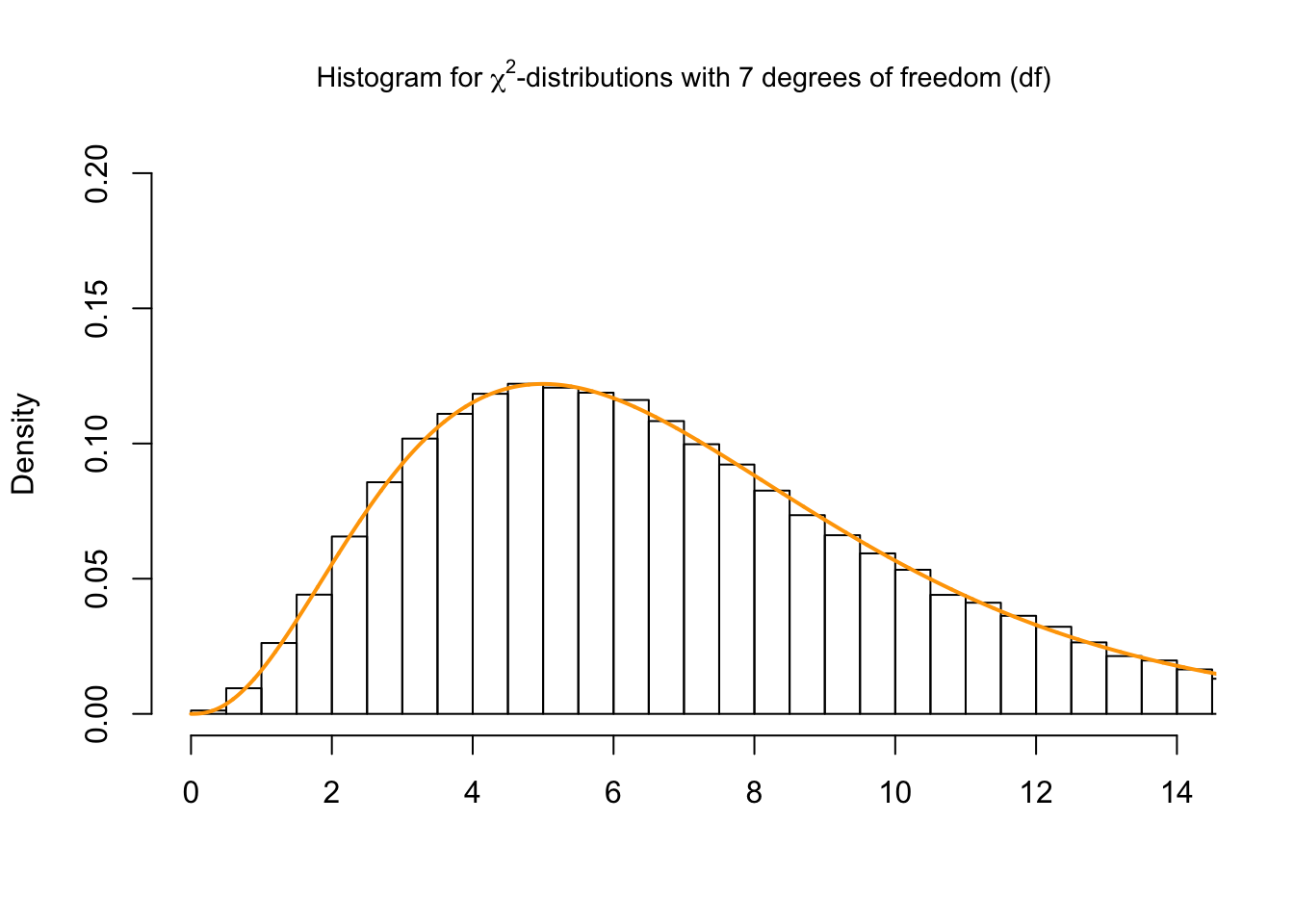The main functions to interact with the $$\chi^2$$-distribution are dchisq(), pchisq(), qchisq(), rchisq(). The dchisq() function gives the density, the pchisq()function gives the distribution function, the qchisq() function gives the quantile function, and the rchisq()function generates random deviates.

We use the dchisq() to calculate the density for the integer values 4 to 8 of a $$\chi^2$$-curve with $$df=7$$.

dchisq(4:8, df = 7)
##  0.11518073 0.12204152 0.11676522 0.10411977 0.08817914

We use the pchisq() to calculate the area under the curve for the interval $$[0,6]$$ and the interval $$[6, \infty)$$ of a $$\chi^2$$-curve with $$df=7$$. Further, we ask R if the sum of the intervals $$[0,6]$$ and $$[6, \infty)$$ sums up to 1.

# interval $[0,6] pchisq(6, df = 7,lower.tail = TRUE) ##  0.4602506 # interval$[6,inf]
pchisq(6, df = 7,lower.tail = FALSE)
##  0.5397494
pchisq(6, df = 7,lower.tail = TRUE) + pchisq(6, df = 7,lower.tail = FALSE) == 1
##  TRUE

We use the qchisq() to calculate the quantile for a given area (= probability) under the curve for a $$\chi^2$$-curve with $$df=7$$ that corresponds to $$q = 0.25, 0.5, 0.75$$ and $$0.999$$. We set lower.tail = TRUE in order the get the area for the interval $$[0, q]$$.

qchisq(0.25, df=7,lower.tail = TRUE)
##  4.254852
qchisq(0.5, df=7,lower.tail = TRUE)
##  6.345811
qchisq(0.75, df=7,lower.tail = TRUE)
##  9.037148
qchisq(0.999, df=7,lower.tail = TRUE)
##  24.32189

We use the rchisq() function to generate 100,000 random values from the $$\chi^2$$-distribution with $$df=7$$. Thereafter we plot a histogram and compare it to the probability density function of the $$\chi^2$$-distribution with $$df=7$$ (orange line).

x <- rchisq(100000, df = 7)
hist(x,
breaks = 'Scott',
freq = FALSE,
xlim = c(0,14),
ylim = c(0,0.2),
xlab = '',
main = (TeX('Histogram for $\\chi^2$-distributions with 7 degrees of freedom (df)')), cex.main=0.9)

curve(dchisq(x, df = 7), from = 0, to = 15, n = 5000, col= 'orange', lwd=2, add = T)